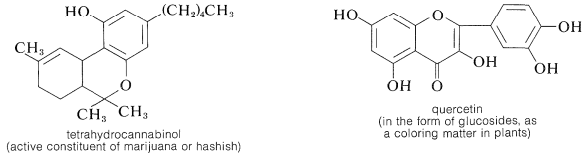# 26.1: Aryl Oxygen Compounds

$$\newcommand{\vecs}{\overset { \rightharpoonup} {\mathbf{#1}} }$$ $$\newcommand{\vecd}{\overset{-\!-\!\rightharpoonup}{\vphantom{a}\smash {#1}}}$$$$\newcommand{\id}{\mathrm{id}}$$ $$\newcommand{\Span}{\mathrm{span}}$$ $$\newcommand{\kernel}{\mathrm{null}\,}$$ $$\newcommand{\range}{\mathrm{range}\,}$$ $$\newcommand{\RealPart}{\mathrm{Re}}$$ $$\newcommand{\ImaginaryPart}{\mathrm{Im}}$$ $$\newcommand{\Argument}{\mathrm{Arg}}$$ $$\newcommand{\norm}{\| #1 \|}$$ $$\newcommand{\inner}{\langle #1, #2 \rangle}$$ $$\newcommand{\Span}{\mathrm{span}}$$ $$\newcommand{\id}{\mathrm{id}}$$ $$\newcommand{\Span}{\mathrm{span}}$$ $$\newcommand{\kernel}{\mathrm{null}\,}$$ $$\newcommand{\range}{\mathrm{range}\,}$$ $$\newcommand{\RealPart}{\mathrm{Re}}$$ $$\newcommand{\ImaginaryPart}{\mathrm{Im}}$$ $$\newcommand{\Argument}{\mathrm{Arg}}$$ $$\newcommand{\norm}{\| #1 \|}$$ $$\newcommand{\inner}{\langle #1, #2 \rangle}$$ $$\newcommand{\Span}{\mathrm{span}}$$$$\newcommand{\AA}{\unicode[.8,0]{x212B}}$$

## Phenols (Arenols) - Physical Properties

Phenols are enols, and enols normally are unstable with respect to the corresponding carbonyl compounds (Section 17-1D). Thus

The situation is different for phenols because of the inclusion of the carbon-carbon double bond into the aromatic ring and the associated aromatic stabilization. Phenol (benzenol) exists exclusively in the enol form:The physical properties of some representative phenols are summarized in Table 26-1. In general, phenols are somewhat more polar than the corresponding saturated alcohols. The magnitudes of the differences are well illustrated by comparison of the physical properties of benzenol and cyclohexanol, shown in Table 26-2. The determining factor appears to be the greater acidity of the phenolic hydroxyl group, which means that, in the undissociated form, the $$\ce{O-H}$$ bond is more strongly polarized as $$\overset{\delta \ominus}{\ce{O}}-\overset{\delta \oplus}{\ce{H}}$$ than for alcohols. Phenols therefore form stronger hydrogen bonds than alcohols, thereby resulting in higher boiling points, higher water solubility, and increased ability to act as solvents for reasonably polar organic molecules.

Table 26-1: Physical Properties of Some Representative PhenolsThe wavelengths of the ultraviolet absorption maxima of the arenols shown in Table 26-1 indicate a considerable effect of substituents on these absorptions, which correspond to the $$200$$-$$\text{nm}$$ and $$255$$-$$\text{nm}$$ absorptions of benzene (Section 22-3B).

Table 26-2: Comparative Physical Properties of Benzenol and CyclohexanolSubstances such as 2-hydroxybenzaldehyde, 2-hydroxybenzoic acid, and 2-nitrobenzenol form intra- rather than intermolecular hydrogen bonds. This effectively reduces intermolecular attraction, thereby reducing boiling points and increasing solubility in nonpolar solvents as compared to the meta and para isomers, which only form intermolecular hydrogen bonds:Figure 26-1 shows the proton resonances of the nitrobenzenol isomers, and you will see that the ortho isomer has the $$\ce{OH}$$ proton resonance at much lower field than either the meta or para isomer. Only for the ortho isomer are the nitro and hydroxyl groups sufficiently close together to form an intramolecular hydrogen bond:Figure 26-1: Nuclear magnetic resonance spectra at $$60 \: \text{MHz}$$ of (a) 2-nitrobenzenol, (b) 3-nitrobenzenol, and (c) 4-nitrobenzenol in ethoxyethane solution (the solvent bands are not shown).

## Synthesis of Phenols

Benzenol and the 2-, 3-, and 4-methylbenzenols (cresols) can be isolated from coal tar (Section 22-11). Benzenol itself is used commercially in such large quantities that alternate methods of preparation are necessary and most of these start with benzene or alkylbenzenes. Direct oxidation of benzene is not satisfactory because benzenol is oxidized more readily than is benzene.

At one time, benzenol was made industrially by sulfonating or chlorinating benzene and then introducing the hydroxyl group by nucleophilic substitution with strong alkali: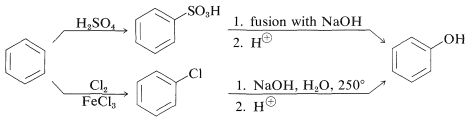Current commercial syntheses of benzenol involve oxidation of methylbenzene or isopropylbenzene (Section 16-9E). Oxidation of isopropylbenzene is economically feasible for the production of benzenol because 2-propanone (acetone) also is a valuable product:A common laboratory procedure converts an aromatic amine to a phenol by way of the arenediazonium salt, $$\ce{ArNH_2} \rightarrow \ce{ArN_2^+} \rightarrow \ce{ArOH}$$ (Section 23-10B).

## Reactions of Phenols Involving the $$\ce{O-H}$$ Bonds

The reactions of the hydroxyl groups of phenols, wherein the $$\ce{O-H}$$ bonds are broken, are similar to those of alcohols. Thus phenols are weak acids ($$K_a = 10^{-10}$$ to $$10^{-8}$$; Table 26-1), intermediate in strength between carboxylic acids and alcohols.

Enols are stronger acids than alcohols because of the increase in electron delocalization in enolate anions as compared to the neutral enols (see Section 15-8A). The stabilization energy of benzenol (Table 21-1) is $$48 \: \text{kcal mol}^{-1}$$, $$5 \: \text{kcal}$$ greater than that of benzene. We can ascribe this increase to delocalization of an unshared electron pair from oxygen:When the $$\ce{OH}$$ proton is removed by a base the resulting anion has even greater stabilization, because the corresponding valence-bond structures do not involve charge separation:We can be confident that substituent groups that stabilize the anion will increase the acidity. Thus 4-nitrobenzenol is about 500 times stronger as an acid than benzenol, because of the increased delocalization of charge to the nitro group: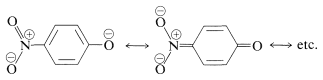It is possible to prepare esters of phenols with carboxylic acid anhydrides or acid halides, and phenyl ethers by reaction of benzenolate anion with halides, sulfate esters, sulfonates, or other alkyl derivatives that react well by the $$S_\text{N}2$$ mechanism:Phenols (like carboxylic acids; Section 24-7C and Table 18-6) are converted to methoxy derivatives with diazomethane:Almost all phenols and enols (such as those of 1,3-diketones) give colors with ferric chloride in dilute water or alcohol solutions. Benzenol itself produces a violet color with ferric chloride, and the methylbenzenols give a blue color. The products apparently are ferric arenolate salts, which absorb visible light to give an excited state having electrons delocalized over both the iron atom and the unsaturated system.

## Reactions of Phenols Involving the $$\ce{C-O}$$ Bonds

In general, it is very difficult to break the aromatic $$\ce{C-O}$$ bond of arenols. Thus concentrated halogen acids do not convert simple arenols to aryl halides, and alkoxyarenes are cleaved with hydrogen bromide or hydrogen iodide in the manner $$\ce{ArO-R}$$ rather than $$\ce{Ar-OR}$$:(Diaryl ethers, such as diphenyl ether, do not react with hydrogen iodide even at $$200^\text{o}$$.) There is no easy way to convert arenols to aryl halides, except where activation is provided by 2- or 4-nitro groups. Thus 2,4-dinitrobenzenol is converted to 1-chloro-2,4-dinitrobenzene with phosphorus pentachloride:An exception to the generalization that $$\ce{C-O}$$ bonds to aromatic systems are difficult both to make and to break is provided by reversible conversion of benzenediols and 1- or 2-naphthalenols to the corresponding amines, usually at elevated temperatures with sodium hydrogen sulfite or an acidic catalyst. The sodium hydrogen sulfite-induced reaction is called the Bucherer reaction: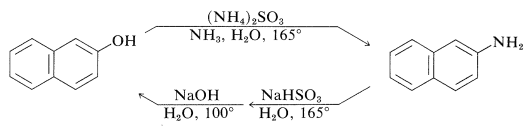These reactions do not work well with simple benzenols because the key step is formation of the keto isomer of the arenol - a process that is unfavorable for simple benzenols.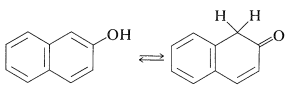$$\tag{26-1}$$

The role of the hydrogen sulfite is participation in a reversible 1,4-addition to the unsaturated ketone to hold it in the ketone form that then is converted to the imine by $$\ce{NH_3}$$ (Section 16-4C) and hence to the arenamine:## Substitution Reactions at the Ring Carbons of Arenols

The electron-rich $$\pi$$-orbital systems of arenols and especially of arenolate ions make these compounds very susceptible to electrophilic substitution. Arenols typically react rapidly with bromine in aqueous solution to substituted the positions ortho or para to the hydroxyl group. Benzenol itself gives 2,4,6-tribromobenzenol in high yield: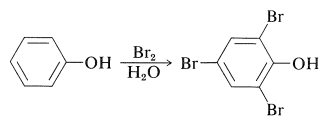Several important reactions of arenols involve aromatic substitution of arenolate ions with carbon electrophiles. In a sense, these reactions are alkylation and acylation reactions as discussed for arenes (Sections 22-4E and 22-4F). In another sense, they are alkylation and acylation reactions of enolate anions and therefore could give rise to products by $$\ce{C}$$- and $$\ce{O}$$-alkylation, or $$\ce{C}$$- and $$\ce{O}$$-acylation (Section 17-4). Thus:In most cases, $$\ce{O}$$-alkylation predominates. However, with 2-propenyl halides either reaction can be made essentially the exclusive reaction by proper choice of solvent. With sodium benzenolate the more polar solvents, such as 2-propanone, lead to 2-propenyloxybenzene, whereas in nonpolar solvents, such as benzene, 2-(2-propenyl)benzenol is the favored product:It should be noted that formation of 2-(2-propenyl)benzenol in nonpolar solvents is not the result of $$\ce{O}$$-propenylation followed by rearrangement, even though the $$\ce{C}$$-propenylation product is thermodynamically more stable. Rearrangement in fact does occur, but at much higher temperatures (above $$200^\text{o}$$) than required to propenylate sodium benzenolate:Such rearrangements are quite general for aryl allyl ethers and are called Claisen rearrangements. They are examples of the pericyclic reactions discussed in Section 21-10D.

The Kolbe-Schmitt reaction produces $$\ce{O}$$- and $$\ce{C}$$-carboxylation through the reaction of carbon dioxide with sodium benzenolate at $$125^\text{o}$$:Sodium benzenolate absorbs carbon dioxide at room temperature to form sodium phenyl carbonate ($$\ce{O}$$-carboxylation) and, when this is heated to $$125^\text{o}$$ under a pressure of several atmospheres of carbon dioxide, it rearranges to sodium 2-hydroxybenzoate (sodium salicylate). However, there is no evidence that this reaction is other than a dissociation-recombination process, in which the important step involves electrophilic attack by carbon dioxide on the aromatic ring of the benzenolate ion ($$\ce{C}$$-carboxylation):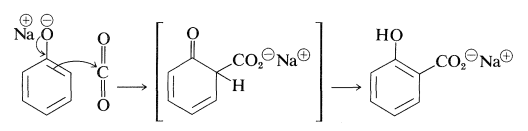With the sodium benzenolate at temperatures of $$125^\text{o}$$ to $$150^\text{o}$$, ortho substitution occurs; at higher temperatures ($$250^\text{o}$$ to $$300^\text{o}$$), particularly with the potassium salt, the para isomer is favored.

The Kolbe-Schmitt reaction is related to enzymatic carboxylations as of $$D$$-ribulose-1,5-diphosphate with carbon dioxide, a key step in photosynthesis (Section 20-9). The overall result is $$\ce{C-C}$$ bond formation by addition of $$\ce{CO_2}$$ to an enolate salt or its enamine equivalent.

In the somewhat related Reimer-Tiemann reaction, sodium benzenolate with trichloromethane in alkaline solution forms the sodium salt of 2-hydroxybenzenecarbaldehyde (salicylaldehyde). The electrophile in this case probably is dichlorocarbene (Section 14-7B):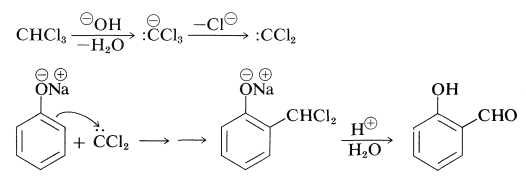Many phenols undergo aldol-like addition reactions with carbonyl compounds in the presence of acids or bases. Thus benzenol reacts with methanal under mild alkaline conditions to form (4-hydroxyphenyl)methanol:The use of this type of reaction in the formation of polymers will be discussed in Chapter 29.

Arenols usually will undergo diazo coupling reactions with aryldiazonium salts at pH values high enough to convert some of the arenol to the more powerfully nucleophilic arenolate anions:However, if the pH is too high, coupling is inhibited because the diazonium salt is transformed into $$\ce{ArN=N-O}^\ominus$$, which is the nonelectrophilic conjugate base of a diazotic acid (Table 23-4).

Arenols can be reduced successfully with hydrogen over nickel catalysts to the corresponding cyclohexanols. A variety of alkyl-substituted cyclohexanols can be prepared in this way:## Oxidation of Arenols

Benzenol can be oxidized to 1,4-benzenedione (para-benzoquinone) by chromic acid. The reaction may proceed by way of phenyl hydrogen-chromate (Section 15-6B) as follows:Oxidation reactions of arenols with other oxidants are complex. Oxidative attack seems to involve, as the first step, removal of the hydroxyl hydrogen to yield a phenoxy radical:The subsequent course depends upon the substituents on the aromatic ring. With 2,4,6-tri-tert-butylbenzenol, the radical is reasonably stable in benzene solution and its presence is indicated by both its dark-blue color and the fact that it adds to 1,3-butadiene:Apparently, dimerization of the above phenoxy radical through either oxygen or the ring is inhibited by the bulky tert-butyl groups. With fewer or smaller substituents, the phenoxy radicals may form dimerization or disproportionation products. Examples of these reactions follow.

Dimerization: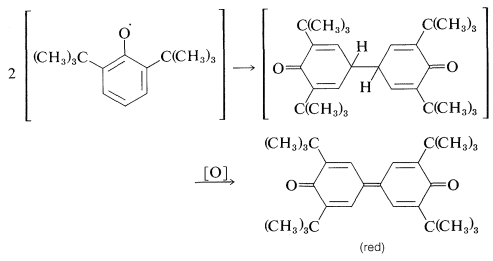Disproportionation:## Arene Polyols

Several important aromatic compounds have more than one arene hydroxyl group. These most often are derivatives of the following dihydric and trihydric arenols, all of which have commonly used (but poorly descriptive) names: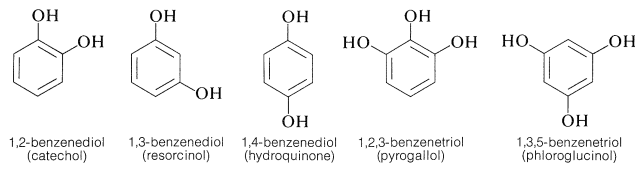All are exceptionally reactive towards electrophilic reagents, particularly in alkaline solution, and all are readily oxidized. The 1,2- and 1,4-benzenediols, but not 1,3-benzenediol, are oxidized to quinones:The preparation of these substances can be achieved by standard methods for synthesizing arenols, but most of them actually are made on a commercial scale by rather special procedures, some of which are summarized as follows:The gallic acid used in the preparation of 1,2,3-benzenetriol can be obtained by microbial degradation of tannins, which are complex combinations of glucose and gallic acid obtained from oak bark and gallnuts. A few other representatives of the many types of naturally occurring derivatives of polyhydric arenols are